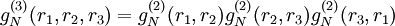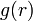# Kirkwood superposition approximation

(diff) ← Older revision | Latest revision (diff) | Newer revision → (diff)
Jump to: navigation, search

Kirkwood 1935 (Eq. 40 \cite{JCP_1935_03_00300}, Eq. 5.6 \cite{PRSA_1946_188_0010})$g_N^{(3)}(r_1,r_2,r_3)=g_N^{(2)}(r_1,r_2)g_N^{(2)}(r_2,r_3)g_N^{(2)}(r_3,r_1)$

It appears that this was used as a basis of a closure for the Kirkwood integral equation \cite{JCP_1935_03_00300} and the Yvon, and Born-Green \cite{PRSA_1946_188_0010} until the work of Morita and Hiroike \cite{PTP_1960_023_1003}.\\ It was pointed out in Ref.s \cite{PR_1952_085_000777,PRSA_1953_216_0203} there is an inconsistency between the pressure and the compressibility equation if this superposition approximation is used to generate$g(r)$.